# Sets (Mathematics class XI)

26. May 2021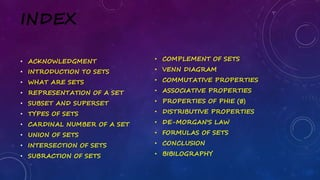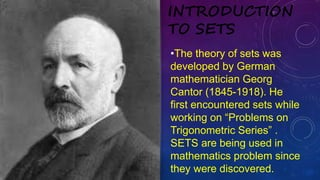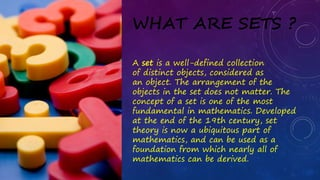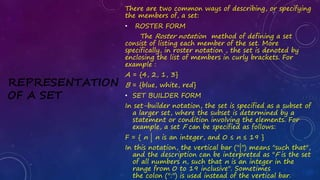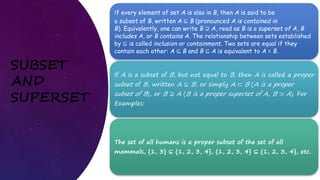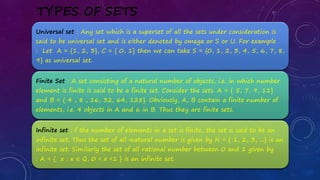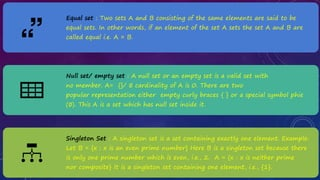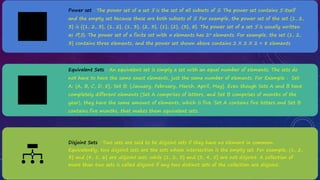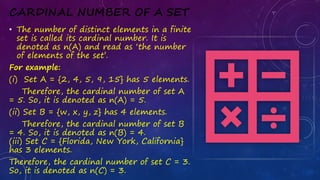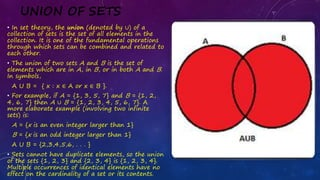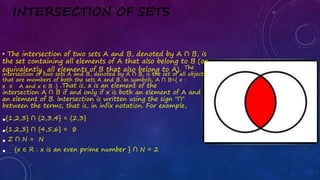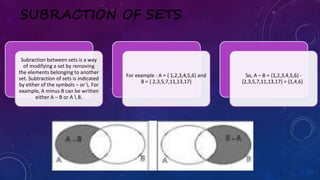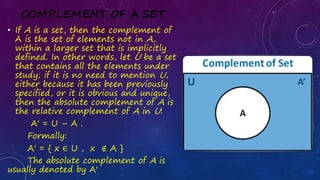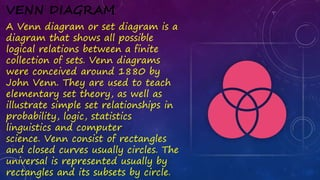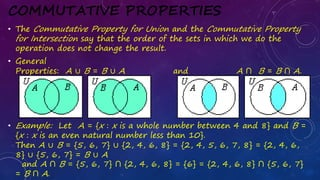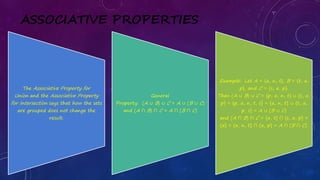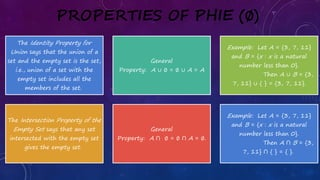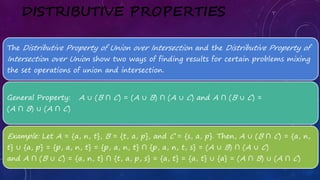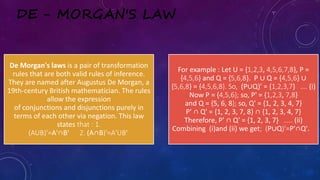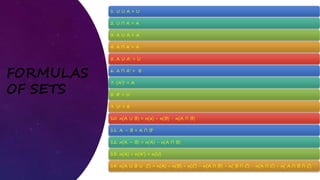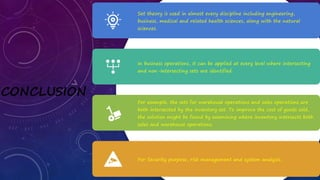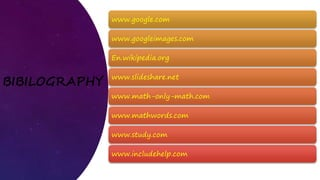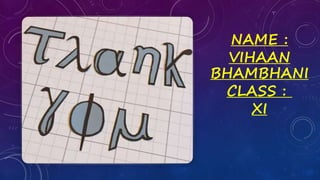1 von 25

### Sets (Mathematics class XI)

• 2. INDEX • ACKNOWLEDGMENT • INTRODUCTION TO SETS • WHAT ARE SETS • REPRESENTATION OF A SET • SUBSET AND SUPERSET • TYPES OF SETS • CARDINAL NUMBER OF A SET • UNION OF SETS • INTERSECTION OF SETS • SUBRACTION OF SETS • COMPLEMENT OF SETS • VENN DIAGRAM • COMMUTATIVE PROPERTIES • ASSOCIATIVE PROPERTIES • PROPERTIES OF PHIE (∅) • DISTRIBUTIVE PROPERTIES • DE-MORGAN'S LAW • FORMULAS OF SETS • CONCLUSION • BIBILOGRAPHY
• 3. ACKNOWLEDGMENT I would like to express my special thanks of gratitude to my teacher Mr. Vaibhav as well as our principal Ms. Veena Sharma who gave me the golden opportunity to do this wonderful project on the topic Sets, which also helped me in doing a lot of Research and i came to know about so many new things I am really thankful to them. Secondly i would also like to thank my parents and friends who helped me a lot in finalizing this project within the limited time frame.
• 4. •The theory of sets was developed by German mathematician Georg Cantor (1845-1918). He first encountered sets while working on “Problems on Trigonometric Series” . SETS are being used in mathematics problem since they were discovered. INTRODUCTION TO SETS
• 5. WHAT ARE SETS ? A set is a well-defined collection of distinct objects, considered as an object. The arrangement of the objects in the set does not matter. The concept of a set is one of the most fundamental in mathematics. Developed at the end of the 19th century, set theory is now a ubiquitous part of mathematics, and can be used as a foundation from which nearly all of mathematics can be derived.
• 6. REPRESENTATION OF A SET There are two common ways of describing, or specifying the members of, a set: • ROSTER FORM The Roster notation method of defining a set consist of listing each member of the set. More specifically, in roster notation , the set is denoted by enclosing the list of members in curly brackets. For example : A = {4, 2, 1, 3} B = {blue, white, red} • SET BUILDER FORM In set-builder notation, the set is specified as a subset of a larger set, where the subset is determined by a statement or condition involving the elements. For example, a set F can be specified as follows: F = { n | n is an integer, and 0 ≤ n ≤ 19 } In this notation, the vertical bar ("|") means "such that", and the description can be interpreted as "F is the set of all numbers n, such that n is an integer in the range from 0 to 19 inclusive". Sometimes the colon (":") is used instead of the vertical bar.
• 7. SUBSET AND SUPERSET If every element of set A is also in B, then A is said to be a subset of B, written A ⊆ B (pronounced A is contained in B). Equivalently, one can write B ⊇ A, read as B is a superset of A, B includes A, or B contains A. The relationship between sets established by ⊆ is called inclusion or containment. Two sets are equal if they contain each other: A ⊆ B and B ⊆ A is equivalent to A = B. If A is a subset of B, but not equal to B, then A is called a proper subset of B, written A ⊊ B, or simply A ⊂ B (A is a proper subset of B), or B ⊋ A (B is a proper superset of A, B ⊃ A). For Examples: The set of all humans is a proper subset of the set of all mammals, {1, 3} ⊆ {1, 2, 3, 4}, {1, 2, 3, 4} ⊆ {1, 2, 3, 4}, etc.
• 8. TYPES OF SETS Universal set : Any set which is a superset of all the sets under consideration is said to be universal set and is either denoted by omega or S or U. For example : Let A = {1, 2, 3}, C = { 0, 1} then we can take S = {0, 1, 2, 3, 4, 5, 6, 7, 8, 9} as universal set. Finite Set : A set consisting of a natural number of objects, i.e. in which number element is finite is said to be a finite set. Consider the sets. A = { 5, 7, 9, 11} and B = { 4 , 8 , 16, 32, 64, 128}. Obviously, A, B contain a finite number of elements, i.e. 4 objects in A and 6 in B. Thus they are finite sets. Infinite set : f the number of elements in a set is finite, the set is said to be an infinite set. Thus the set of all-natural number is given by N = { 1, 2, 3, ...} is an infinite set. Similarly the set of all rational number between 0 and 1 given by : A = { x : x ∈ Q, 0 < x <1 } is an infinite set.
• 9. Equal set : Two sets A and B consisting of the same elements are said to be equal sets. In other words, if an element of the set A sets the set A and B are called equal i.e. A = B. Null set/ empty set : A null set or an empty set is a valid set with no member. A= {}/ ∅ cardinality of A is 0. There are two popular representation either empty curly braces { } or a special symbol phie (∅). This A is a set which has null set inside it. Singleton Set : A singleton set is a set containing exactly one element. Example: Let B = {x : x is an even prime number} Here B is a singleton set because there is only one prime number which is even, i.e., 2. A = {x : x is neither prime nor composite} It is a singleton set containing one element, i.e., {1}.
• 10. Power set : The power set of a set S is the set of all subsets of S. The power set contains S itself and the empty set because these are both subsets of S. For example, the power set of the set {1, 2, 3} is {{1, 2, 3}, {1, 2}, {1, 3}, {2, 3}, {1}, {2}, {3}, ∅}. The power set of a set S is usually written as P(S). The power set of a finite set with n elements has 2n elements. For example, the set {1, 2, 3} contains three elements, and the power set shown above contains 2 X 2 X 2 = 8 elements Equivalent Sets : An equivalent set is simply a set with an equal number of elements. The sets do not have to have the same exact elements, just the same number of elements. For Example : Set A: {A, B, C, D, E}, Set B: {January, February, March, April, May}. Even though Sets A and B have completely different elements (Set A comprises of letters, and Set B comprises of months of the year), they have the same amount of elements, which is five. Set A contains five letters and Set B contains five months, that makes them equivalent sets. Disjoint Sets : Two sets are said to be disjoint sets if they have no element in common. Equivalently, two disjoint sets are the sets whose intersection is the empty set. For example, {1, 2, 3} and {4, 5, 6} are disjoint sets, while {1, 2, 3} and {3, 4, 5} are not disjoint. A collection of more than two sets is called disjoint if any two distinct sets of the collection are disjoint.
• 11. CARDINAL NUMBER OF A SET • The number of distinct elements in a finite set is called its cardinal number. It is denoted as n(A) and read as ‘the number of elements of the set’. For example: (i) Set A = {2, 4, 5, 9, 15} has 5 elements. Therefore, the cardinal number of set A = 5. So, it is denoted as n(A) = 5. (ii) Set B = {w, x, y, z} has 4 elements. Therefore, the cardinal number of set B = 4. So, it is denoted as n(B) = 4. (iii) Set C = {Florida, New York, California} has 3 elements. Therefore, the cardinal number of set C = 3. So, it is denoted as n(C) = 3.
• 12. UNION OF SETS • In set theory, the union (denoted by ∪) of a collection of sets is the set of all elements in the collection. It is one of the fundamental operations through which sets can be combined and related to each other. • The union of two sets A and B is the set of elements which are in A, in B, or in both A and B. In symbols, A U B = { x : x ∈ A or x ∈ B }. • For example, if A = {1, 3, 5, 7} and B = {1, 2, 4, 6, 7} then A ∪ B = {1, 2, 3, 4, 5, 6, 7}. A more elaborate example (involving two infinite sets) is: A = {x is an even integer larger than 1} B = {x is an odd integer larger than 1} A U B = {2,3,4,5,6, . . . } • Sets cannot have duplicate elements, so the union of the sets {1, 2, 3} and {2, 3, 4} is {1, 2, 3, 4}. Multiple occurrences of identical elements have no effect on the cardinality of a set or its contents.
• 13. INTERSECTION OF SETS • The intersection of two sets A and B, denoted by A ∩ B, is the set containing all elements of A that also belong to B (or equivalently, all elements of B that also belong to A). The intersection of two sets A and B, denoted by A ∩ B, is the set of all objects that are members of both the sets A and B. In symbols, A ∩ B={ x : x ∈ A and x ∈ B .} .That is, x is an element of the intersection A ∩ B if and only if x is both an element of A and an element of B. Intersection is written using the sign "∩" between the terms; that is, in infix notation. For example, •{1,2,3} ∩ {2,3,4} = {2,3} •{1,2,3} ∩ {4,5,6} = ∅ • Z ∩ N = N • {x ∈ R : x is an even prime number } ∩ N = 2
• 14. SUBRACTION OF SETS Subraction between sets is a way of modifying a set by removing the elements belonging to another set. Subtraction of sets is indicated by either of the symbols – or . For example, A minus B can be written either A – B or A B. For example : A = { 1,2,3,4,5,6} and B = { 2,3,5,7,11,13,17} So, A – B = {1,2,3,4,5,6} - {2,3,5,7,11,13,17} = {1,4,6}
• 15. COMPLEMENT OF A SET • If A is a set, then the complement of A is the set of elements not in A, within a larger set that is implicitly defined. In other words, let U be a set that contains all the elements under study; if it is no need to mention U, either because it has been previously specified, or it is obvious and unique, then the absolute complement of A is the relative complement of A in U: A' = U – A . Formally: A' = { x ∈ U , x ∉ A } The absolute complement of A is usually denoted by A'
• 16. VENN DIAGRAM A Venn diagram or set diagram is a diagram that shows all possible logical relations between a finite collection of sets. Venn diagrams were conceived around 1880 by John Venn. They are used to teach elementary set theory, as well as illustrate simple set relationships in probability, logic, statistics linguistics and computer science. Venn consist of rectangles and closed curves usually circles. The universal is represented usually by rectangles and its subsets by circle.
• 17. COMMUTATIVE PROPERTIES • The Commutative Property for Union and the Commutative Property for Intersection say that the order of the sets in which we do the operation does not change the result. • General Properties: A ∪ B = B ∪ A and A ∩ B = B ∩ A. • Example: Let A = {x : x is a whole number between 4 and 8} and B = {x : x is an even natural number less than 10}. Then A ∪ B = {5, 6, 7} ∪ {2, 4, 6, 8} = {2, 4, 5, 6, 7, 8} = {2, 4, 6, 8} ∪ {5, 6, 7} = B ∪ A and A ∩ B = {5, 6, 7} ∩ {2, 4, 6, 8} = {6} = {2, 4, 6, 8} ∩ {5, 6, 7} = B ∩ A.
• 18. ASSOCIATIVE PROPERTIES The Associative Property for Union and the Associative Property for Intersection says that how the sets are grouped does not change the result. General Property: (A ∪ B) ∪ C = A ∪ (B ∪ C) and (A ∩ B) ∩ C = A ∩ (B ∩ C) Example: Let A = {a, n, t}, B = {t, a, p}, and C = {s, a, p}. Then (A ∪ B) ∪ C = {p, a, n, t} ∪ {s, a, p} = {p, a, n, t, s} = {a, n, t} ∪ {t, a, p, s} = A ∪ (B ∪ C) and (A ∩ B) ∩ C = {a, t} ∩ {s, a, p} = {a} = {a, n, t} ∩ {a, p} = A ∩ (B ∩ C).
• 19. PROPERTIES OF PHIE (∅) The Identity Property for Union says that the union of a set and the empty set is the set, i.e., union of a set with the empty set includes all the members of the set. General Property: A ∪ ∅ = ∅ ∪ A = A Example: Let A = {3, 7, 11} and B = {x : x is a natural number less than 0}. Then A ∪ B = {3, 7, 11} ∪ { } = {3, 7, 11}. The Intersection Property of the Empty Set says that any set intersected with the empty set gives the empty set. General Property: A ∩ ∅ = ∅ ∩ A = ∅. Example: Let A = {3, 7, 11} and B = {x : x is a natural number less than 0}. Then A ∩ B = {3, 7, 11} ∩ { } = { }.
• 20. DISTRIBUTIVE PROPERTIES The Distributive Property of Union over Intersection and the Distributive Property of Intersection over Union show two ways of finding results for certain problems mixing the set operations of union and intersection. General Property: A ∪ (B ∩ C) = (A ∪ B) ∩ (A ∪ C) and A ∩ (B ∪ C) = (A ∩ B) ∪ (A ∩ C) Example: Let A = {a, n, t}, B = {t, a, p}, and C = {s, a, p}. Then, A ∪ (B ∩ C) = {a, n, t} ∪ {a, p} = {p, a, n, t} = {p, a, n, t} ∩ {p, a, n, t, s} = (A ∪ B) ∩ (A ∪ C) and A ∩ (B ∪ C) = {a, n, t} ∩ {t, a, p, s} = {a, t} = {a, t} ∪ {a} = (A ∩ B) ∪ (A ∩ C)
• 21. DE - MORGAN'S LAW De Morgan's laws is a pair of transformation rules that are both valid rules of inference. They are named after Augustus De Morgan, a 19th-century British mathematician. The rules allow the expression of conjunctions and disjunctions purely in terms of each other via negation. This law states that : 1. (AUB)'=A'∩B' 2. (A∩B)'=A'UB' For example : Let U = {1,2,3, 4,5,6,7,8}, P = {4,5,6} and Q = {5,6,8}. P ∪ Q = {4,5,6} ∪ {5,6,8} = {4,5,6,8}. So, (P∪Q)' = {1,2,3,7} …. (i) Now P = {4,5,6}; so, P' = {1,2,3, 7,8} and Q = {5, 6, 8}; so, Q' = {1, 2, 3, 4, 7} P' ∩ Q' = {1, 2, 3, 7, 8} ∩ {1, 2, 3, 4, 7} Therefore, P' ∩ Q' = {1, 2, 3, 7} ….. (ii) Combining (i)and (ii) we get; (P∪Q)'=P'∩Q'.
• 22. FORMULAS OF SETS 1. U U A = U 2. U ∩ A = A 3. A U A = A 4. A ∩ A = A 5. A U A' = U 6. A ∩ A' = ∅ 7. (A')' = A 8. ∅' = U 9. U' = ∅ 10. n(A U B) = n(a) + n(B) - n(A ∩ B) 11. A – B = A ∩ B' 12. n(A – B) = n(A) - n(A ∩ B) 13. n(A) + n(A') = n(U) 14. n(A U B U C) = n(A) + n(B) + n(C) - n(A ∩ B) - n( B ∩ C) - n(A ∩ C) + n( A ∩ B ∩ C)
• 23. CONCLUSION Set theory is used in almost every discipline including engineering, business, medical and related health sciences, along with the natural sciences. In business operations, it can be applied at every level where intersecting and non-intersecting sets are identified. For example, the sets for warehouse operations and sales operations are both intersected by the inventory set. To improve the cost of goods sold, the solution might be found by examining where inventory intersects both sales and warehouse operations. For Security purpose, risk management and system analysis.